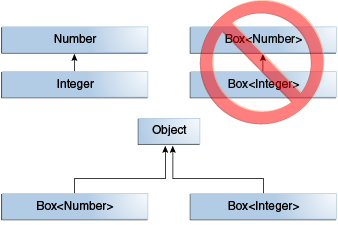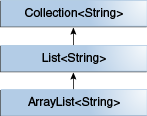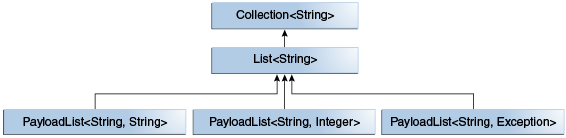# # 泛型、继承和子类型

``````Object someObject = new Object();
Integer someInteger = new Integer(10);
someObject = someInteger;   // OK
``````
1
2
3

``````public void someMethod(Number n) { /* ... */ }

someMethod(new Integer(10));   // OK
someMethod(new Double(10.1));   // OK
``````
1
2
3
4

``````Box<Number> box = new Box<Number>();
``````
1
2
3

``````public void boxTest(Box<Number> n) { /* ... */ }
``````
1TIP

## # 泛型类和子类型``````interface PayloadList<E,P> extends List<E> {
...
}
``````
1
2
3
4

PayloadList 的以下参数是`List <String>`的子类型：

``````PayloadList<String,String>